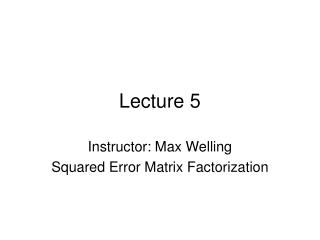# Lecture 5 - PowerPoint PPT PresentationDownload PresentationLecture 5

Lecture 5
Download Presentation## Lecture 5

- - - - - - - - - - - - - - - - - - - - - - - - - - - E N D - - - - - - - - - - - - - - - - - - - - - - - - - - -
##### Presentation Transcript

1. Lecture 5 Instructor: Max Welling Squared Error Matrix Factorization

2. total of +/- 400,000,000 nonzero entries (99% sparse) movies (+/- 17,770) users (+/- 240,000) Remember User Ratings • We are going to “squeeze out” the noisy, un-informative information from • the matrix. • In doing so, the algorithm will learn to only retain the most valuable information • for predicting the ratings in the data matrix. • This can then be used to more reliably predict the entries that were not rated.

3. K x movies (+/- 17,770) Squeezing out Information total of +/- 400,000,000 nonzero entries (99% sparse) movies (+/- 17,770) users (+/- 240,000) K users (+/- 240,000) “K” is the number of factors, or topics.

4. Interpretation star-wars = [10,3,-4,-1,0.01] K • Before, each movie was characterized by a signature • over 240,000 user-ratings. • Now, each movie is represented by a signature of • K “movie-genre” values (or topics, or factors) • Movies that are similar are expected to have similar • values for their movie genres. movies (+/- 17,770) users (+/- 240,000) K • Before, users were characterized by their ratings over all movies. • Now, each user is represented by his/her values over movie-genres. • Users that are similar have similar values for their movie-genres.

5. Dual Interpretation • Interestingly, we can also interpret the factors/topics as • user-communities. • Each user is represented by its “participation” in K • communities. • Each movie is represented by the ratings of these • communities for the movie. • Pick what you like! I’ll call them topics or factors from now on. K movies (+/- 17,770) users (+/- 240,000) K

6. The Algorithm B A • In an equation, the squeezing boils down to: • We want to find A,B such that we can reproduce the observed ratings as • best as we can, given that we are only given K topics. • This reconstruction of the ratings will not be perfect (otherwise we over-fit) • To do so, we minimize the squared error (Frobenius norm):

7. Prediction • We train A,B to reproduce the observed ratings only and we ignore all the • non-rated movie-user pairs. • But here come the magic: after learning A,B, the product AxB will have filled • in values for the non-rated movie-user pairs! • It has implicitly used the information from similar users and similar movies • to generate ratings for movies that weren’t there before. • This is what “learning” is: we can predict things from we haven’t seen before • by looking at old data.

8. The Algorithm • We want to minimize the Error. Let’s take the gradients again. • We could now follow the gradients again: • But wait, • how do we ignore the non-observed entries ? • for netflix, this will actually be very slow, how can we make this practical ?

9. Stochastic Gradient Descent • First we pick a single observed movie-user pair at random: Rmu. • The we ignore the sums over u,m in the exact gradients, and do an update: • The trick is that although we don’t follow the exact gradient, on average • we do move in the correct direction.

10. Stochastic Gradient Descent stochastic updates full updates (averaged over all data-items) • Stochastic gradient descent does not converge to the minimum, but “dances” • around it. • To get to the minimum, one needs to decrease the step-size as one get closer • to the minimum. • Alternatively, one can obtain a few samples and average predictions over them • (similar to bagging).

11. Weight-Decay • Often it is good to make sure the values in A,B do not grow too big. • We can make that happen by adding weight decay terms which keep them small. • Small weights are often better for generalization, but this depends on how • many topics, K, you choose. • The simplest weight decay results in: • But a more aggressive variant is to replace

12. Incremental Updates • One idea is to train one factor at a time. • It’s fast • One can monitor performance on held out test data • A new factor is trained on the residuals of the model up till now. • Hence, we get: • If you fit slow (i.e. don’t go to convergence, weight decay, shrinkage) results are better.

13. Pre-Processing • We are almost ready for implementation. • But we can make a head-start if we first remove some “obvious” structure • from the data, so the algorithm doesn’t have to search for it. • In particular, you can subtract the user and movie means where you • ignore missing entries. • Nmu is the total number of observed movie-user pairs. • Note that you can now predict missing entries by simply using:

14. Homework • Subtract the movie and user means from the netflix data. • Check theoretically and experimentally that the both user and movie means are 0. • make a prediction for the quiz data and submit it to netflix. • (your RMSE should be around 0.99) • Implement the stochastic matrix factorization and submit to netflix. • (you should get around 0.93)